# WA Arbitrary Path Reconstruction VI

LabVIEW 2014 Advanced Signal Processing Toolkit Help

Edition Date: June 2014

Part Number: 372656C-01

»View Product InfoDownload Help (Windows Only)

Owning Palette: Discrete Wavelet VIs

Requires: Advanced Signal Processing Toolkit

Reconstructs a signal with subband coefficients. Wire data to the path coef input to determine the polymorphic instance to use or manually select the instance.

Example

Use the pull-down menu to select an instance of this VI.

 Select an instance WA Arbitrary Path Reconstruction (Waveform)WA Arbitrary Path Reconstruction (Array)

## WA Arbitrary Path Reconstruction (Waveform)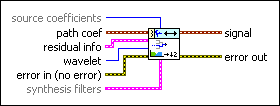source coefficients specifies the coefficients for reconstructing the time waveform.

 0 All coefficients—Reconstructs the original input signal from path coef and residual info. 1 Path coefficients (default)—Reconstructs the bandpass signal from path coef. 2 Residual coefficients—Reconstructs the residual signal after bandpass filtering from residual info.path coef specifies the coefficients of the subband that the path input of the WA Arbitrary Path Decomposition VI defines.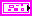residual info specifies the information about the complementary subbands at each level. The residual paths describe these complementary subbands. For example, when path is 101, the residual paths include path 0, path 11, and path 100. This VI uses the complementary subbands information for signal reconstruction.coef specifies the coefficients on the residual paths. For example, when path is 101, coef includes the coefficients on path 0, path 11, and path 100.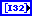length specifies the length of the coefficients on the residual paths and the signal length. For example, when path is 101, length includes the signal length and the lengths on path 0, path 11, and path 100.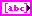residual path specifies the residual paths.wavelet specifies the wavelet type of the discrete wavelet analysis. The default is db02. The options include two types: orthogonal (Haar, Daubechies (dbxx), Coiflets (coifx), Symmlets (symx)) and biorthogonal (Biorthogonal (biorx_x), including FBI (bior4_4 (FBI))), where x indicates the order of the wavelet. wavelet must be the same type as the wavelet type you selected in the WA Arbitrary Path Decomposition VI to compute path coef and residual info. If you used the analysis filters input of the WA Arbitrary Path Decomposition VI to specify wavelet, ignore this input and use the synthesis filters input of this VI to specify the synthesis filters of the wavelet.error in describes error conditions that occur before this node runs. This input provides standard error in functionality.synthesis filters specifies the coefficients of the lowpass synthesis filters and the highpass synthesis filters for the wavelet you specify. If you specify a value for synthesis filters, this VI ignores the settings in the wavelet input. You can use the Wavelet Design Express VI to design the analysis filters and the corresponding synthesis filters.lowpass specifies the coefficients of the lowpass synthesis filter, which this VI uses to filter the interpolated approximation coefficients in the wavelet reconstruction.highpass specifies the coefficients of the highpass synthesis filter, which this VI uses to filter the interpolated detail coefficients in the wavelet reconstruction.signal returns the reconstructed signal.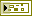error out contains error information. This output provides standard error out functionality.

## WA Arbitrary Path Reconstruction (Array)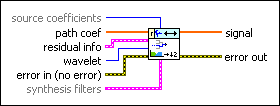source coefficients specifies the coefficients for reconstructing the time waveform.

 0 All coefficients—Reconstructs the original input signal from path coef and residual info. 1 Path coefficients (default)—Reconstructs the bandpass signal from path coef. 2 Residual coefficients—Reconstructs the residual signal after bandpass filtering from residual info.path coef specifies the coefficients of the subband that the path input of the WA Arbitrary Path Decomposition VI defines.residual info specifies the information about the complementary subbands at each level. The residual paths describe these complementary subbands. For example, when path is 101, the residual paths include path 0, path 11, and path 100. This VI uses the complementary subbands information for signal reconstruction.coef specifies the coefficients on the residual paths. For example, when path is 101, coef includes the coefficients on path 0, path 11, and path 100.length specifies the length of the coefficients on the residual paths and the signal length. For example, when path is 101, length includes the signal length and the lengths on path 0, path 11, and path 100.residual path specifies the residual paths.wavelet specifies the wavelet type of the discrete wavelet analysis. The default is db02. The options include two types: orthogonal (Haar, Daubechies (dbxx), Coiflets (coifx), Symmlets (symx)) and biorthogonal (Biorthogonal (biorx_x), including FBI (bior4_4 (FBI))), where x indicates the order of the wavelet. wavelet must be the same type as the wavelet type you selected in the WA Arbitrary Path Decomposition VI to compute path coef and residual info. If you used the analysis filters input of the WA Arbitrary Path Decomposition VI to specify wavelet, ignore this input and use the synthesis filters input of this VI to specify the synthesis filters of the wavelet.error in describes error conditions that occur before this node runs. This input provides standard error in functionality.synthesis filters specifies the coefficients of the lowpass synthesis filters and the highpass synthesis filters for the wavelet you specify. If you specify a value for synthesis filters, this VI ignores the settings in the wavelet input. You can use the Wavelet Design Express VI to design the analysis filters and the corresponding synthesis filters.lowpass specifies the coefficients of the lowpass synthesis filter, which this VI uses to filter the interpolated approximation coefficients in the wavelet reconstruction.highpass specifies the coefficients of the highpass synthesis filter, which this VI uses to filter the interpolated detail coefficients in the wavelet reconstruction.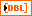signal returns the reconstructed signal.error out contains error information. This output provides standard error out functionality.

## Example

Refer to the Engine Knocking Detection VI in the labview\examples\Wavelet Analysis\WAApplications directory for an example of using the WA Arbitrary Path Reconstruction VI.

Not Helpful